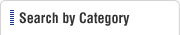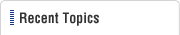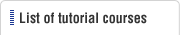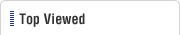HOME  > Low Cost Automation Tutorial  > #227 Weight Reduction Techniques - 13: Weight Reduction Techniques - Cross-Section Shape for Lightweight and High-Strength Structure
Low Cost Automation Tutorial

#227 Weight Reduction Techniques - 13: Weight Reduction Techniques - Cross-Section Shape for Lightweight and High-Strength Structure

Category : Low-cost automation and materials
March11, 2016

We introduced an example of "lightweight and strong structural members" using bamboo in the previous section. The strong bend-resistance achieved by the hollow architecture is described by a technical term "second moment of area". This section explains the second moment of area in an easy-to-understand manner.

(1)Terms describing the strength of structural members

The strength can be described by the following terms depending on what it refers to.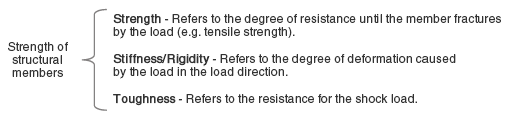Bamboo trees have large "stiffness" because of their hollow cylinder with nodes. In addition, they also have high "toughness" for their tissue structure being more densely distributed as they become closer to the epidermis. Since they excel in both stiffness and toughness, we can conclude that bamboo trees are the lightweight yet strong structural member.

(2)Cross-section shape and bending strength

The second moment of area (written as an alphabet "I") is a technical term that refers to bend resistance for the bending force applied to bar-shaped structural members. This second moment of area can be modified by the cross-section shape of structural members. Therefore, it is possible to design a lightweight and highly strong structure by adopting the optimal cross-section shape.

 Cross-section shape Cross-section area (A) Second moment of area (I) Computational comparison of the same area Square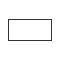Width: b Height: h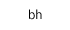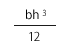When b=31.4 and h=10, A=314. At this time, I=2,617. Circle (circular cylinder)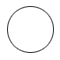Radius: r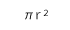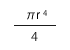When r=10, A=314. At this time, I=7,850. Hollow cylinder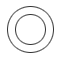Outer radius: R Inner radius: r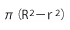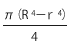When R=15 and r=11.2, A=314. At this time, I=27,388.

The formula of second moment of area (I) for various cross-section shapes is listed in the MISUMI FA Standard Catalog Technology Data: Area, Gravity Center, and Second Moment of Area (P1-1890).